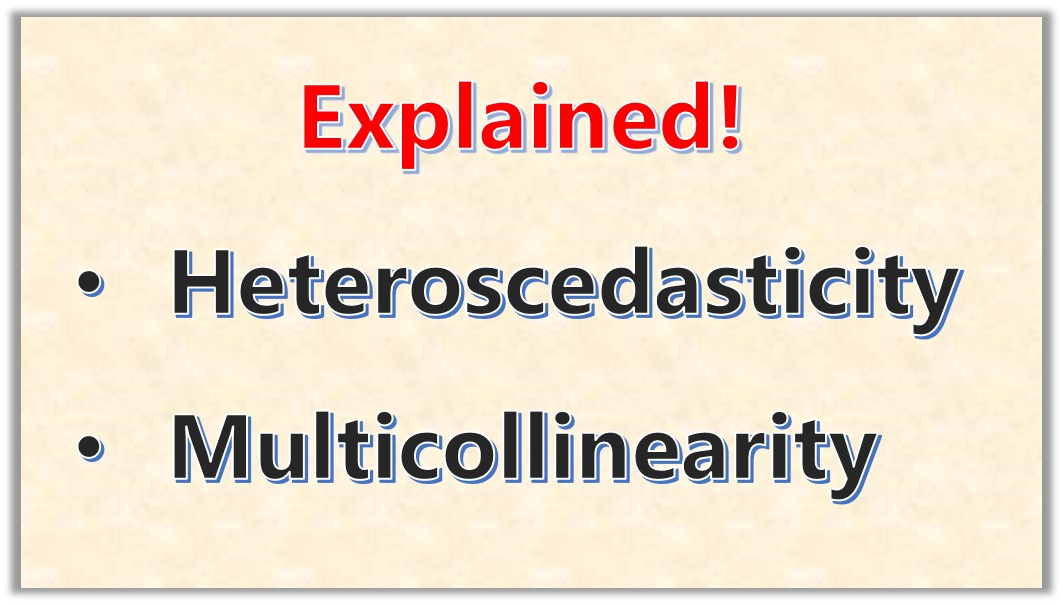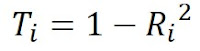# What is Heteroscedasticity and Multicolinearity in Regression Analysis?Hello! Good to see you. I’ll be very happy to explain to you these two important concepts

• Multicolinearity
• Heteroscedasticity

These are big words, but trust me they are very easy to understand. Normally, these two terms are not directly related but they are two situations that create some problem during regression analysis.

So just as the two words are difficult to pronounce, they also make analysis procedure difficult!

As you know, I normally explain math and statistics concepts in a simple way to allow you clearly understand it and be able to present it in your own words during a quiz or an exam.

What is Heteroscedasticity?
Heteroscedasticity  means unequal scatter. This means that the  variability(or scatter) of a variable is unequal accross the range of values of the other variable that is used to predict it.
It is a systematic change in spread of the residual over the range of the measured values. This is illustrated in Figure 1.0The Problem of Heteroscedasticity
In Linear Regression, it is assumed that the measured error (or residual) between the measured value and the regression line maintains a constant variance (homoscedasticity).
But in the case of heteroscedasticity, the residuals have an unequal variance which makes the regression assumption unreliable.

What is Multicolinearity?
To understand this term, you need to keep in mind that in multivariate linear regression,  the objective is to examine the effect of more than one independent variable,
x1, x2,… xk on a dependent variable Y.
So the regression function would be as shown below:
Where
Y is the dependent variable

x1, x2,… xk are the independent variables
Ɛ is the error term

Now, these independent  variables not only affects the dependent variable but also either a strengthening or weakening effect on each other. Therefore, multicolinearity means the linear correlation relationship between two or more independent variables.

Effects of Multicolinearity
Multicolinearity has unpleasant effects which are highlighted below:

• the independent variables may assume each other’s role
• the effect of the independent variables on the dependent variable cannot be distinguished
• estimation of the regression coefficients is rendered unreliable
• In some cases, analysis becomes very difficult to perform

Measure of Multicolinearity
There are three metrics used to examine the effects of multicolinearity. They are:

• VIF (Variation Inflation Factor)
• Tolerance
• Condition Indices

Variation Inflation Factor (VIF): The VIF shows the actual variance of the estimated coefficient of the ith variable, how many variables after this variable would be without the effect of muticolinearity. Same is calculated for the other variables.

if the VIF is between 1 and 2 , then there is strong multicolinearity. If betwee 2 to 5, then it is very. For values above 5, there is very high multicolinearity.

VIF is given by the formulaTolerance: This is given as the inverse of the Variation Inflation factor.  A low tolerance value indicates an increasing multicolinearityCondition Index: This is a measure of the relative amount of variation associated with an eigenvalue. Large value of the condition index indicates a high degree of collinearity.